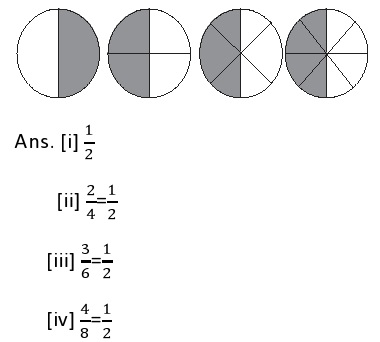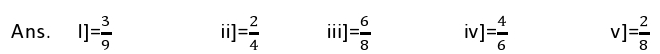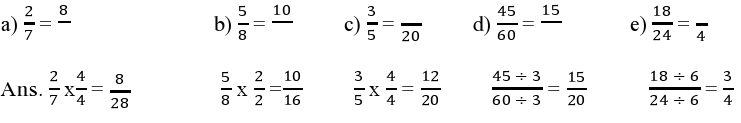EXERCISE 7.3:

1). Write the following are all these fractions equivalent?
a)YES, ALL OF THESES ARE EQUIVALENT FRACTIONS2). Write the fraction and pair up the equivalent fraction from each rowPairing up

[a] = [ii], [b] = [iv], [c] = [i], [d] = [v], [e] = [vi]

3). Replace — in each of the following by the correct number:4). Find the equivalent fraction of 3/5 having
a) Denominator 20
Ans. 3/5 = (3×4) / (5×4) = 12/20
b) Numerator 9
Ans. (3×3) / (5×3) =  9/15
c) Denominator 30
Ans. (3×6) / (5×6) =  18/30
d) Numerator 27
Ans. (3×9) / (5×9) = 27/45

5). Find the equivalent fraction of 36/48 with
a) Numerator 9
Ans. 36/48 = (36 ÷ 4) / (48÷4) = 9/12
b) Denominator 4
Ans. (36 ÷ 12) / (48 ÷ 12) = 3/4

6). Check whether the given fraction are equivalent:
a) 5/9 , 30/54
Ans. (5 × 6) / (9 × 6) = 30/54
Therefore,5 / (9 ) , 30/54 are equivalent
b) (3 /10) , (12/ 50)
Ans. (3 × 5) / (10 × 5) = 15/50
Therefore 3 /10 , 12 /50 are not equivalent
c). (7/13) , (5/11)
Ans. (7 × 11)/(13 × 11) , (5 × 13)/(11 × 13) = 77 /143 , 65 / 143
Therefore, 7 /13 , 5 /11 are not equivalent

7). Reduce the following fraction to simplest form
i) 48/60
Ans. The HCF of 48 and 60 is 12 therefore (48 ÷  12) / (60÷12) = 4/5
ii) 150/60
Ans. The HCF of 150 and 60 is 30 therefore (150 ÷ 30) / (60÷30) = 5/2
iii) 84/98
Ans. The HCF of 84 and 98 is 14 therefore (84 ÷ 14) / (98÷14) = 6/7
iv) 12/52
Ans. The HCF of 12 and 52 is 4 therefore (12 ÷ 4)  /(52÷4) = 3/13
v) 7/28
Ans. The HCF of 7 and 28 is 7 therefore (7 ÷ 7) / (28÷7) = 1/4

8). Ramesh had 20 pencils, Sheelu had 50 pencils and Jamal had 80 pencils. After 4 months, Ramesh used up 10 pencils, Sheelu used up 25 pencils and Jamaal used up 40 pencils. What fraction did each use up check if each has used up on equal fraction of their pencil?
Ans.
Ramesh : total pencil = 20

Used pencil = 10
Required fraction = 10/20 = 1/2
Sheelu: total pencil = 50
Used pencil = 25
Required fraction = 25/50 = 1/2
Jamaal: total pencil = 80
Used pencil = 25
Required fraction = 40/80 = 1/2
Since all of them used half of their pencil therefore each one used up equal fraction of their pencil.

9). Match the equivalent fractions and write two more for each:
i)250/400                                   a) 2/3
ii)180/200                                  b) 2/3
iii)660/990                                c) 1/2
iv)180/360                                 d) 5/8
v)220/550                                  e) 9/10
Ans.
i) 250/400 = 5/(8 ) , 15/24 ,10/16           d) 5/8
ii) 180/200 = 9/10 , 18/20 , 27/10           e) 9/10
iii) 660/990 = 2/3 ,4/6 ,6/9                      a) 2/3
iv)180/360 = 1/2 , 2/4 ,3/6                       c) 1/2
v) 220/550 = 2/5 , 4/10 , 6/15                  b) 2/5